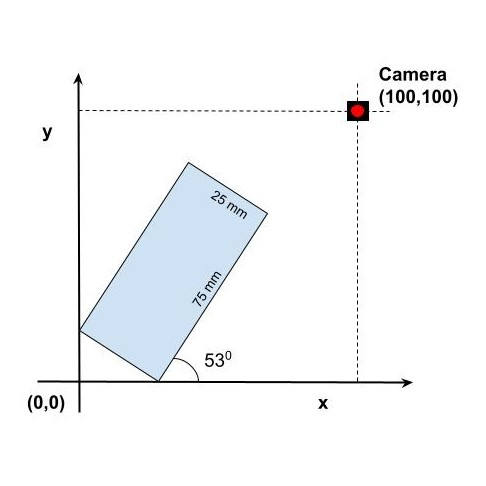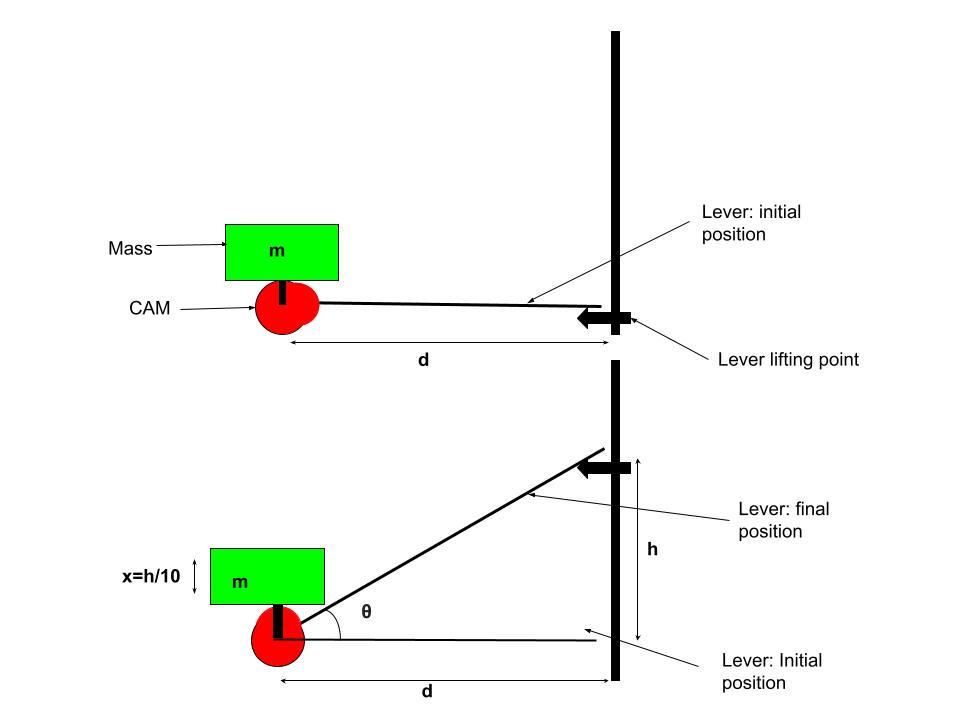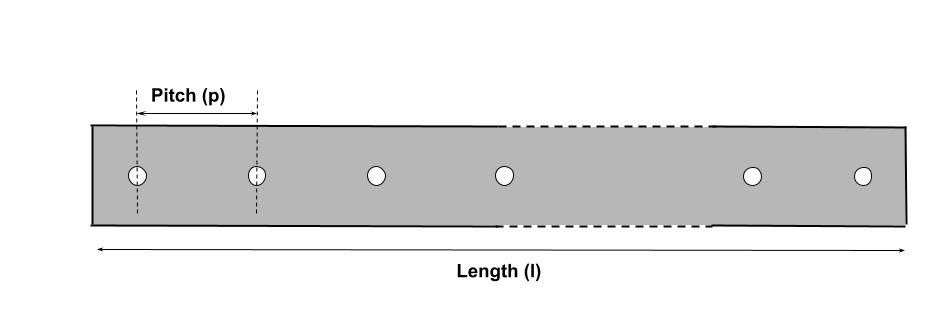Welcome to Online evaluation for Mechanical Design Engineering role @ Morphle Labs
The test has 1 hour window from 2pm-3pm Sunday, 7th Nov, 2021

The test has 3 questions. Answer as many questions as you can.. good luck!

# Question 1A Rectangular plate (denoted as slide in the image) of dimensions 25mmx75mm is placed in a 2D space with the x and y axis as shown in the above picture. It is attached to an xy linear actuation system. The origin is fixed. Two corners of the plate are on the x and y axes when the actuation system is at (0,0). Plate is tilted at 53𝆩 with respect to x at all times.

# Question 2A CAM drives a mass up and down when we turn the lever using the lever lifter.
The ratio of the vertical displacement between the mass and the Lever lifting point is 1:10.
In other words, if the lever lifter moves up 'h' distance from a lever horizontal position as shown in the figure, the mass will move up h/10 distance.One way to write this relationship is; x=h/10, where x is the vertical displacement of the mass.
Write another way we can write the above equation using 'theta'.

# Question 3In abowe image,
If l = 2375 mm & p = 37 mm